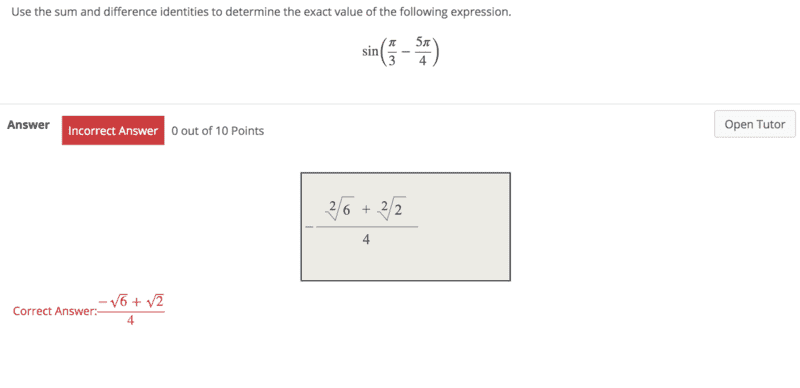• opus
In summary, I was given an incorrect solution to a problem and was corrected by someone else. The solution is -\frac{6+2}{4}

Gold Member

## Homework Statement

For the solution to a given problem, in the second to last step I had:
##-\frac{\sqrt 6}{4} + \frac{\sqrt 2}{4}##

I stated next that the solution was ##-\frac{\sqrt{6}+\sqrt{2}}{4}##

I was told this was incorrect and that the correct solution is ##\frac{-\sqrt{6}+\sqrt{2}}{4}##

## The Attempt at a Solution

Could someone explain this error to me? Is it not true that ##-\frac{a}{b}=\frac{-a}{b}=\frac{a}{-b}##?
Or is this case different because I have an expression on the top, not just a single variable?

Screenshot attached for reference.opus said:

## Homework Statement

For the solution to a given problem, in the second to last step I had:
##-\frac{\sqrt 6}{4} + \frac{\sqrt 2}{4}##

I stated next that the solution was ##-\frac{\sqrt{6}+\sqrt{2}}{4}##

I was told this was incorrect and that the correct solution is ##\frac{-\sqrt{6}+\sqrt{2}}{4}##

## The Attempt at a Solution

Could someone explain this error to me? Is it not true that ##-\frac{a}{b}=\frac{-a}{b}=\frac{a}{-b}##?
##-\frac{a}{b}=\frac{-a}{b}## is true. So ##-\frac{\sqrt 6}{4} =\frac{-\sqrt 6}{4}##and ##\frac{-\sqrt 6}{4}+\frac{\sqrt2}{4}=\frac{-\sqrt 6+\sqrt2}{4}##

•opus
opus said:

## Homework Statement

For the solution to a given problem, in the second to last step I had:
##-\frac{\sqrt 6}{4} + \frac{\sqrt 2}{4}##

I stated next that the solution was ##-\frac{\sqrt{6}+\sqrt{2}}{4}##

I was told this was incorrect and that the correct solution is ##\frac{-\sqrt{6}+\sqrt{2}}{4}##

## The Attempt at a Solution

Could someone explain this error to me? Is it not true that ##-\frac{a}{b}=\frac{-a}{b}=\frac{a}{-b}##?
Or is this case different because I have an expression on the top, not just a single variable?

You are guilty of sloppy use (or non-use) of parentheses:
$$-\frac{a}{b} + \frac{c}{b}$$
means that you subtract the fraction ##\frac{a}{b}## from the fraction ##\frac{c}{b}##, and since they have the same denominator, you can combine the numerators as ##c-a##. That is, you get
$$\frac{c-a}{b},$$
which can be written as $$\frac{-a + c}{b}.$$
If you write
$$-\frac{a+c}{b}$$
that means $$- \left( \frac{a+c}{b} \right),$$
which was not what you started with.

Remember the priority order of mathematical operations: reading from left to right, parentheses have precedence over everything, then powers have precedence over multiplication or division, and these have precedence over addition and subtraction. Therefore, when we parse the expression ##-\frac{a}{b} + \frac{c}{b}##, we do "##-##" first, then ##a/b## then "##+##", then ##\frac{c}{b}##. In other words, had we used parentheses we would have had ##(- \frac{a}{b}) + (\frac{c}{b})##.

•opus
Ahh I see. So by pulling that negative all the way out front, I negated the entire expression which is not equal to what I had. The negative needed to stay with the ##\sqrt 6##

•FactChecker
opus said:
Ahh I see. So by pulling that negative all the way out front, I negated the entire expression which is not equal to what I had. The negative needed to stay with the ##\sqrt 6##

Yes, exactly, because in ##-\frac{a+c}{b}## the division has prioity over the first "##-##", so first we do the fraction, then we change its sign.

See, eg.,
http://www.purplemath.com/modules/orderops.htm
or
https://en.wikipedia.org/wiki/Order_of_operations

•opus
Excellent, thanks guys.

•berkeman
opus said:
Ahh I see. So by pulling that negative all the way out front, I negated the entire expression which is not equal to what I had. The negative needed to stay with the ##\sqrt 6##
Yes. If you still want to put a negative in front of the entire thing, you can put another '-' in front of the ##\sqrt 2##. That would give it a "double negative" and so leave it positive. ##-\frac {\sqrt 6} 4 + \frac {\sqrt 2} 4 = - \frac { \sqrt 6 - \sqrt 2 } 4 ##

•opus
Thank you!